# Catch up on the latest AI articles# Can Language Models Be Grounded In Non-verbal Conceptual Space?

3 main points
✔️ Can language models perform grounding to real-world-like conceptual spaces with only a few examples?
✔️Test whether we can learn mappings between 2D and 3D color spaces on the grid and the language space
✔️ Achieve some non-random accuracy with GPT-3

written by Roma PatelEllie Pavlick
(Submitted on 29 Sep 2021)code：The images used in this article are from the paper, the introductory slides, or were created based on them.

## first of all

Large-scale pre-trained language models such as GPT-3 have shown excellent results on a variety of natural language tasks.

In addition to answering questions and producing fluent sentences, such models show a good grasp of the structure of concepts in the linguistic space, including the ability to reason about objects and properties that they have not physically observed.

In this article, we present challenging work that addresses the question of whether language models trained only on textual data can learn grounding to conceptual spaces, for example, real-world spatial information. (This paper has been Accepted (Poster) in ICLR2022)

## experimental setup

### model

GPT-2 and GPT-3 models are used in the experiments. The number of parameters consists of 124M, 355M, 774M, 1.5B, and 175B. The behavior is controlled by giving a small number of examples instead of updating the parameters of these models.

More specifically, after giving the model an example task in the form of "World:~~" for the question and "Answer:~~" for the answer, we can make the model generate an answer to the question by giving it a new question and the data "Answer:" (this is called in-context learning or called few-shot prompting).

### Grounded concept domain

The experiment will address three issues related to concepts that are connected (grounded) to the real world, such as orientation in two-dimensional space and color in RGB space, as illustrated in the following figure.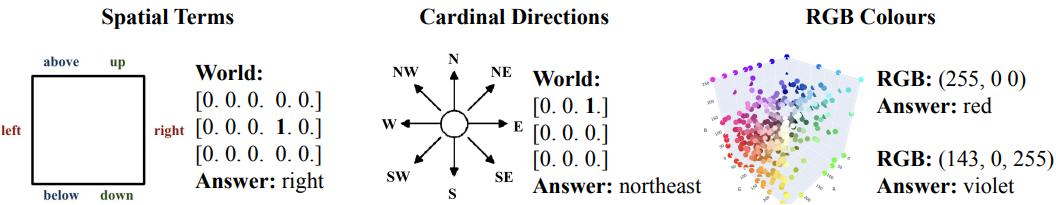The description of each is as follows.

Spatial Terms

In this problem, we consider six spatial concepts (left, right, up, down, top, bottom). Specifically, a two-dimensional space with multiple "0s" and a "1" arranged in a grid is given to the language model as a question ("World:~"), and the language model is asked to answer where the "1" is located.

Cardinal Directions

In this task, you will represent east, west, north, south, south, and the eight orientations in between in a grid two-dimensional space.

Similar to Spatial Terms, but includes compositional terms (compound terms?) like "northeast". The difference is that it contains

RGB Colours

Using the RGB367 color dataset, in which color names (red, cyan, forest green, etc.) are represented by RGB codes, we consider the representation of colors in 3D space.

For example, if "RGB:(255,0,0)" is given as a question, "Answer: red" is the correct answer.

However, the language model used in the experiments (GPT-x) is trained on the CommonCrawl corpus, so it is possible that these domains were encountered during pre-training.

For example, many tables on the Internet show the correspondence between (255,0,0) and "red".

To avoid that the model succeeds only by simply storing such data, we perform an additional rotation process on the task described above.

For example, here is an example of rotation in the RGB color domain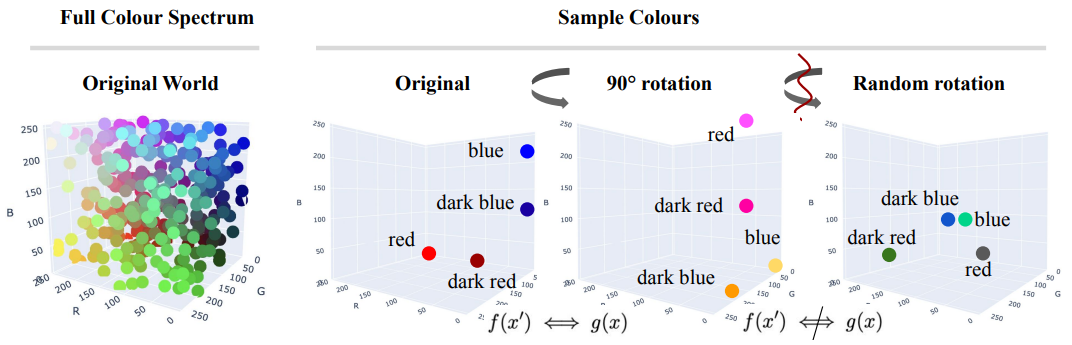If the language model can grasp the structural relationships between concepts in the space (color in this example), it should be able to solve the task even if rotational processing is performed that does not break the spatial structure.

Conversely, if concepts in the space are assigned randomly, the performance should decrease to a random level. In our experiments, we evaluate the model for the original world, as well as for worlds rotated by 90°, 180°, 270°, and random worlds.

If the model successfully maps concepts in the desired way, we expect to obtain high performance in the original/rotated world and low performance in the random world.

The evaluation indicators are as follows

• Top-1 Accuracy: The accuracy is 1 if the ground truth or its substring (word) sequence is in the first $n$ tokens of the generated answer. For example, if the ground truth is "deep Tuscan red", the accuracy is 1 if the model answer is "Tuscan red" or "red", and 0 if it is "deep red", "wine" or "vermilion". In the appendix of the original paper, there is an experiment in which the accuracy is calculated using only full matches instead of partial matches.
• Top-3 Accuracy: An accuracy of 1 is obtained if the correct answer exists in any of the three most probable answer sequences from 1 to 3 in the model.
• Grounding Distance: Used to evaluate the degree of model error. If the answer produced by the model is a word in the domain (e.g. "pink" in the color domain), the Euclidean distance between two points in that space is used for evaluation. If the answer is outside the domain, we set a very large value for this metric.

For grounding distance, an example of the results of this metric for ground truth and predicted results are shown below.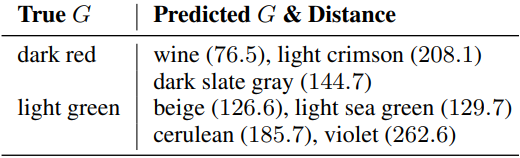This table shows the actual predictions of the GPT-3 model. For example, if "wine" is predicted when the ground truth is "dark red", the grounding distance is 76.5.

baseline

Set the following two random baselines as baselines.

• R-IV (Random In-Vocabulary): selects a random token from the entire vocabulary of the model. (Naturally, it shows almost zero performance on all tasks.)
• R-ID (Random In-Domain): Randomly select a word (e.g. color) in the domain.

## experimental results

### On the ability to generalize to the unseen world

Among the three tasks, we will experiment with Spatial Terms and Cardinal Directions. An example of an input to the model is as follows.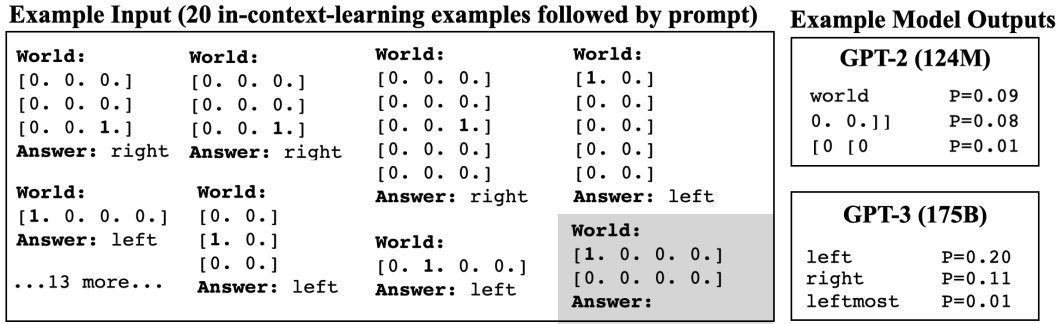Here, the concepts (direction and orientation) included in each task at the time of evaluation are all included in the data previously given to the model. In other words, there is no such thing as "up" or "down" appearing in the evaluation, even though only "right" and "left" appeared in the training data. The data is given to the model in the form of 20 examples of concepts consisting of eight directions or pairs of up, down, left, and right.

The results are as follows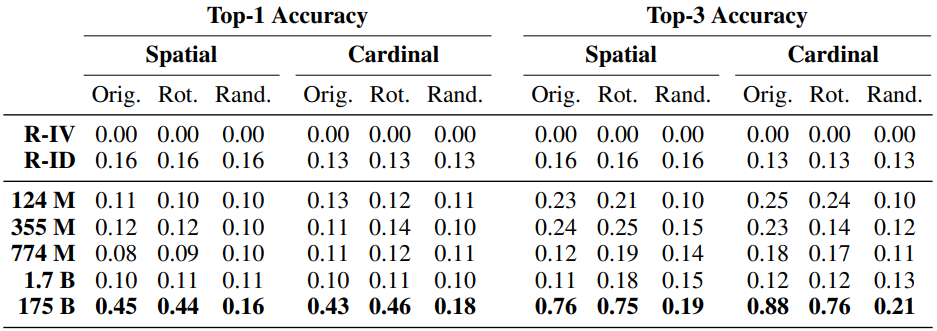The model with the largest number of parameters (GPT-3) performs with a top-1 accuracy of about 0.45 for both the original and rotated worlds, with performance degrading for the random world. This is a desirable result, although the accuracy is not high, and the model generalizes successfully to two-dimensional space to some extent.

On the other hand, for the other models, the performance is below even the R-ID setting, which means that they almost fail to learn.

### On the ability to generalize to unseen concepts

Next, we experiment with tasks that include RGB Colours. In this case, an example input to the model would be as follows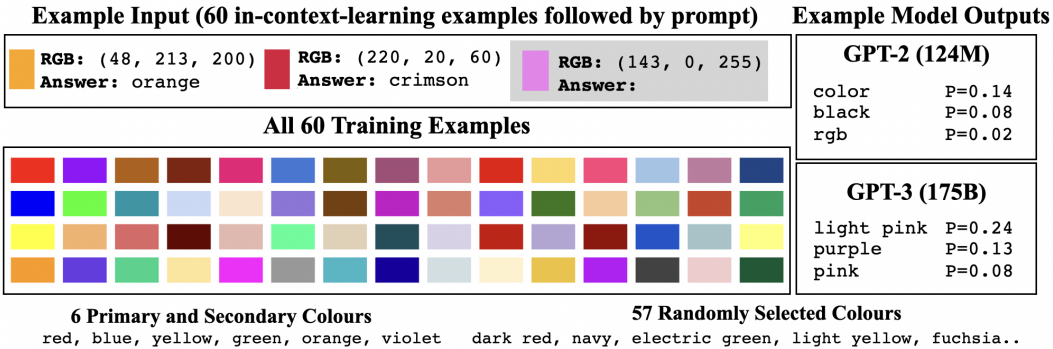Here, some of the concepts (direction, orientation, color) included in each task at the time of evaluation are not included in the data previously provided to the model. In other words, there are cases where only "right" and "left" appear during training, but "up" and "down" appear during evaluation.

More specifically, for Spatial Terms and Cardinal Directions, the data given 20 examples in advance contain $n-1$ concepts, and the data containing the remaining one is used during evaluation.

Due to a large number of concepts in RGB Colours, 60 examples are given in the pre-given data.

The results are as follows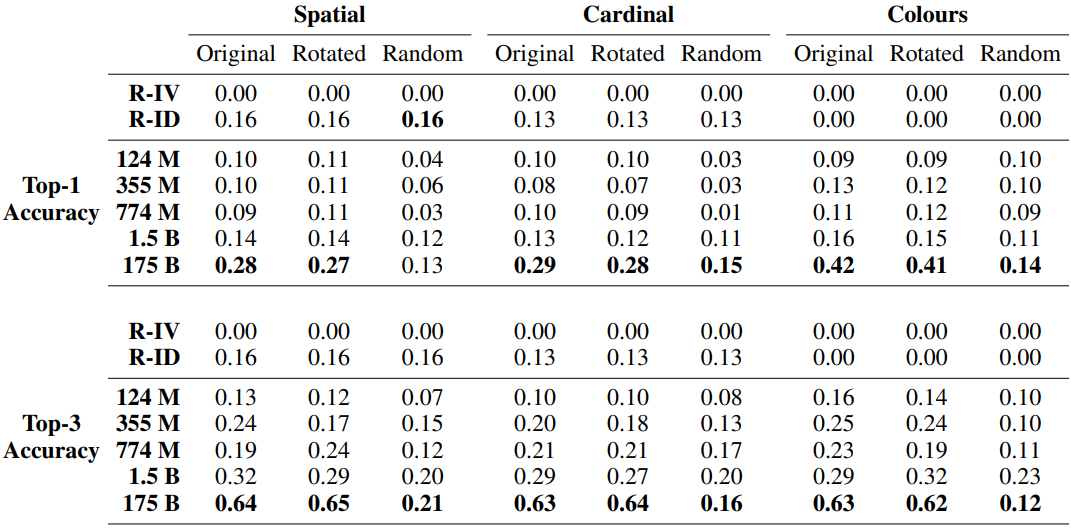As before, the model with the largest number of parameters (GPT-3) performed better than random. In particular, the RGB Colours task shows a Top-1 accuracy of over 40%, which is desirable given the difficulty of the task. The results of the Top-1 and Top-3 accuracy when calculating the Top-1 and Top-3 accuracy with only complete matches instead of partial matches are as follows.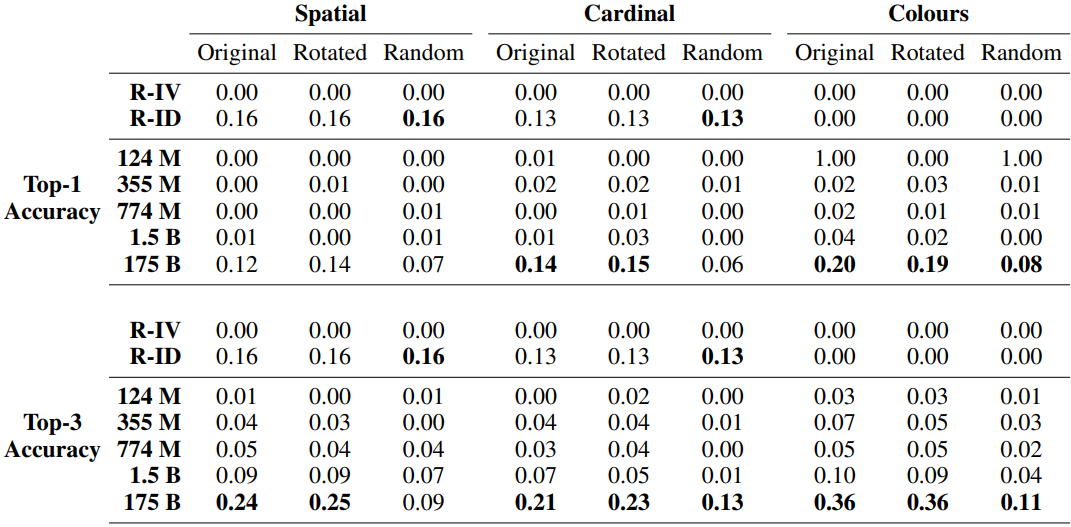The results for the average grounding distance for the RGB Colours task are also shown below.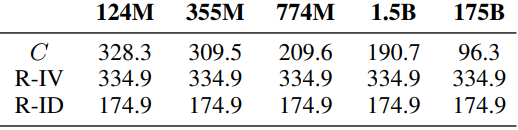The largest model performed significantly better than random (the smaller the number the better). In addition, when asked whether the model's predictions were concepts within its domain (e.g., if it was the Cardinal Directions task, would any of the eight orientations have been generated), the smallest model yielded 53% and the largest model 98% of the responses within its domain.

## summary

In this article, I introduced a study that deals with a very interesting problem related to grounding, which addresses whether language models trained only on textual data can ground into (real-world-like) conceptual spaces, such as three-dimensional color spaces or two-dimensional spaces The following is an example of the use of the

Experimental results showed some success in this attempt for GPT-3, which has a very large number of parameters.

This result may suggest an interesting possibility that language models trained only on text may be able to successfully map or ground the language space to a different conceptual space, depending on very few examples.

If you have any suggestions for improvement of the content of the article,